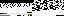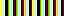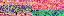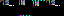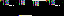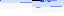```EVR.Level.Delegate = function(level)
{
this.level = level;
this.evr = level.evr;
this.prompt = level.prompt;
this.countdown = level.countdown;
}
EVR.Level.Delegate.prototype.enter = function()
{
var level = this.level;
var prompt = this.prompt;
if (level.states[LEVEL_STATE_WAITING])
{
prompt.remove();
this.countdown.start();
prompt.set_text(LEVEL_PAUSE_PROMPT_TEXT);
}
else if (level.states[LEVEL_STATE_RACING])
{
level.pause();
}
else if (level.states[LEVEL_STATE_SUMMARIZING])
{
level.show_records();
}
else if (level.states[LEVEL_STATE_FINISHED])
{
prompt.set_finish_text();
prompt.toggle();
}
}
EVR.Level.Delegate.prototype.up = function()
{
if (this.path.playing)
{
this.racer.move(-1);
}
}
EVR.Level.Delegate.prototype.down = function()
{
if (this.path.playing)
{
this.racer.move(1);
}
}
EVR.Level.Delegate.prototype.sprint = function()
{
this.path.sprint();
}
EVR.Level.Delegate.prototype.cancel_sprint = function()
{
this.path.sprinting = false;
}
EVR.Level.Delegate.prototype.restart = function()
{
var level = this.level;
if (level.states[LEVEL_STATE_PAUSED] || level.states[LEVEL_STATE_FINISHED])
{
}
}
{
var level = this.level;
if (level.states[LEVEL_STATE_PAUSED] || level.states[LEVEL_STATE_FINISHED])
{
}
}
EVR.Level.Delegate.prototype.next_level = function()
{
var level = this.level;
if (level.states[LEVEL_STATE_FINISHED])
{
var id = parseInt(level.id);
var evr = this.evr;
if (level.next_level_is_available())
{
}
else if (id == LEVEL_LIMIT && level.is_clear())
{
}
}
}
EVR.Level.Delegate.prototype.toString = function()
{
return "[object EVR.Level.Delegate]";
}
```
```EVR.Level.Clock = function(level)
{
EVR.Graphic.call(
this.height = CLOCK_HEIGHT;
this.build();
this.append();
}
EVR.Level.Clock.prototype = new EVR.Graphic;
EVR.Level.Clock.prototype.build = function()
{
this.set_proportions(CLOCK_WIDTH, this.height);
this.set_opacity(CLOCK_OPACITY);
this.set_color(CLOCK_BACKGROUND);
this.set_text("00:00.000", CLOCK_FONT, CLOCK_TEXT_COLOR, CLOCK_TEXT_SIZE);
this.css.fontWeight = CLOCK_FONT_WEIGHT;
this.css.letterSpacing = CLOCK_LETTER_SPACING;
this.set_line_height();
this.css.overflow = "hidden";
this.time = new EVR.Time();
}
EVR.Level.Clock.prototype.set_line_height = function()
{
this.css.lineHeight = this.get_dimensions() + "px";
}
EVR.Level.Clock.prototype.start = function()
{
var current = this;
this.timestamp = +new Date;
this.interval = window.setInterval(
function() {
current.measure();
}, CLOCK_INTERVAL);
}
EVR.Level.Clock.prototype.measure = function()
{
var timestamp = +new Date;
var difference = timestamp - this.timestamp;
this.timestamp = timestamp;
this.text.set(this.time.get_formatted());
}
EVR.Level.Clock.prototype.stop = function()
{
if (!!this.interval)
{
window.clearInterval(this.interval);
this.interval = null;
this.measure();
}
}
EVR.Level.Clock.prototype.toggle = function()
{
if (!!!this.interval)
{
this.start();
}
else
{
this.stop();
}
}
EVR.Level.Clock.prototype.place = function()
{
EVR.Graphic.prototype.place.call(this);
this.set_coordinates([null, y])
}
EVR.Level.Clock.prototype.draw = function()
{
EVR.Graphic.prototype.draw.call(this);
this.set_line_height();
}
EVR.Level.Clock.prototype.toString = function()
{
return "[object EVR.Level.Clock]";
}
```
```<?php
require_once "../account/get_user_path.php";
\$GLOBALS["PROGRESS_FILE_NAME"] = "progress";
\$path = account\get_user_path() . \$GLOBALS["PROGRESS_FILE_NAME"];
\$progress = trim(file_get_contents(\$path));
if (isset(\$_GET["clear"]) && \$_GET["clear"] > \$progress)
{
\$progress++;
file_put_contents(\$path, \$progress);
}
echo \$progress;
```
```<?php
require_once "../account/get_user_path.php";
\$GLOBALS["FILE_NAME"] = "expert";
{
\$id = \$_GET["id"];
\$levels = get_list();
if (!in_array(\$id, \$levels))
{
\$levels[] = \$id;
}
file_put_contents(build_path(), implode(" ", \$levels));
}
function get_list()
{
\$raw = file_get_contents(build_path());
if (\$raw == "")
{
return array();
}
return explode(" ", \$raw);
}
function build_path()
{
return account\get_user_path() . \$GLOBALS["FILE_NAME"];
}
```
44.210.21.70
44.210.21.70
44.210.21.70

June 23, 2019